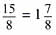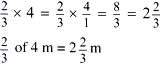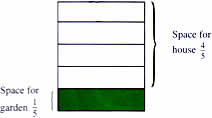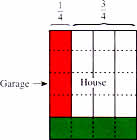Thursday , July 7 2022# NCERT 5th Class (CBSE) Mathematics: Fractions

(b) 5×3/8 = ?

5×3/8 = 5/1×3/8

5/1×3/8 = 15/8(c) 5/6×1 = ?

5/6×1 = 5

(d) 0×3/7 = ?

0×3/7 = 0

(e) In a class of 40 children, 3/4 use the school bus to come to school. How many children use the school bus?

To find 3/4 of 40, you can multiply.

3/4×40 ?

3/4×40 = 3/4×40/1 = 120/4 = 30

Answer: 30 students in the class come to school by the school bus.

(f) What is 2/3 of 4 meters### B. Multiplying Fractions by Fractions

(a) This is Venkatesh’s plot land. He wants to build a house on it.

He wants to keep 1/5 of the land as a garden in front of the house.Of the portion meant for the house, he keeps 1/4 as garage space for his car. The rest of the space is for building the house.

What part of the total land does Venkatesh use to build the house?

From the diagram we can see that Venkatesh uses 12/20 part of the total land to build his house.To be able to find this answer without a diagram, we must solve it as follows.

Venkatesh uses 3/4 of 4/5 of the total land to build his house.

3/4 of 4/5 = ?

3/4 of 4/5 is the same as 3/4×4/5

3/4×4/5 = ?

Step 1. Multiply the numerators: 3/4×4/5 = 12

Step 2. Multiply the denominators: 3/4×4/5 = 12/20

Step 3. Reduce to the lowest term: 3/4×4/5 = 12/20 = 3/5

Venkatesh uses 3/5 of the land to build his house.

## चुनौती हिमालय की 5th NCERT CBSE Hindi Rimjhim Ch 18

चुनौती हिमालय की 5th Class NCERT CBSE Hindi Book Rimjhim Chapter 18 प्रश्न: लद्दाख जम्मू-कश्मीर राज्य में …

### One comment

1.I need worksheet for Class 5th Mathematics.